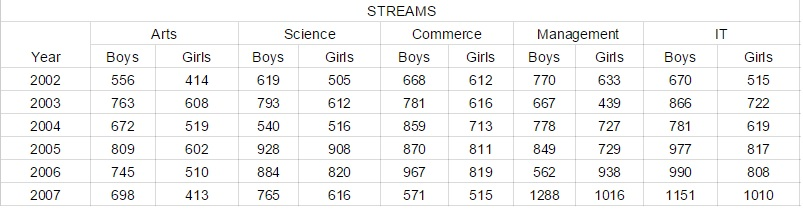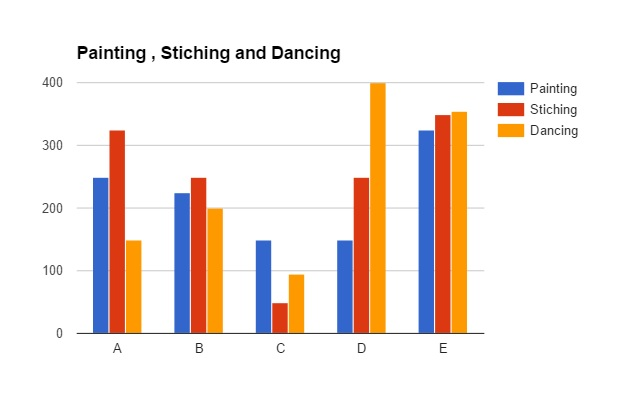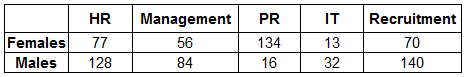# Expected Data Interpretation Sets for IBPS PO PDF

0
301

## Expected Data Interpretation Sets for IBPS PO PDF

Download Top-15 Banking Exams Expected Data Interpretation Sets PDF. Banking Exams Expected Data Interpretation Sets based on asked questions in previous exam papers very important for the Banking  exams.

Take a IBPS PO free mock test

Instructions

Study the following table carefully to answer the question that follows:

NUMBER OF BOYS AND GIRLS IN FIVE STREAMS OF A COLLEGE OVER THE YEARSQuestion 1: What is the total number of boys, for all the streams together, in the year 2004?

a) 4148

b) 3630

c) 4433

d) 3247

e) None of these

Question 2: The number of boys in Art stream in the year 2004 is approximately what per cent of the total number of boys for all the years together in Art stream ?

a) 27

b) 34

c) 08

d) 39

e) 16

Question 3: What is the ratio of the total number of boys to the total numbers of girls from all the stream together for the year 2007 ?

a) 2:3

b) 14:13

c) 52:49

d) 213: 170

e) None of these

Question 4: What is the ratio of the total number of boys to the total number of girls, in the Management stream for all the years together ?

a) 9:8

b) 71:86

c) 91:83

d) 27:23

e) None of these

Question 5: What is the average number of Girls from Commerce stream for the given years?

a) 681

b) 675

c) 618

d) 657

e) None of these

Instructions

Study the information carefully to answer the following questions:

In an organisation consisting of 750 employees the ratio of Males to Females is 8:7 respectively. All the employees work in five different departments viz. HR, Management, PR, IT and Recruitment. 16 per cent of the Females work in Management Department. 32 per cent of Males are in HR Department. One-fifth of the Females are in the Department of Recruitment. The ratio of Males to Females in the Management Department is 3:2 respectively. 20 per cent of the total numbers of employees are in PR Department.Females working in Recruitment are 50 per cent of the Males working in the same Department.8 per cent of the Males are in IT Department.The remaining Males are in PR Department.22 per cent of the Females work in HR Department and the remaining Females are working in IT Department

Question 6: What is the total number of females working in the IT and Recruitment Department together ?

a) 147

b) 83

c) 126

d) 45

e) None of these

Question 7: What is the number of females working in the HR Department ?

a) 77

b) 70

c) 56

d) 134

e) None of these

Question 8: Number of males working in HR Department forms approximately what per cent of total number of the employees in the organisation ?

a) 20

b) 28

c) 32

d) 9

e) 17

Question 9: Number of males working in PR Department forms what per cent of the numbers of Females working in the same Department ? (rounded off to two digits after decimal)

a) 22.98

b) 16.68

c) 11.94

d) 6.79

e) 27.86

Question 10: What is the total number of employees working in the Management Department ?

a) 128

b) 77

c) 210

d) 140

e) None of these

Instructions

Study the graph carefully to answer the question that follow:
Number of Girls Enrolled in Different Hobby
Classes in various Institutes in a yearQuestion 11: What is the respective ratio of total number of girls enrolled in painting in the Institutes A and C together to those enrolled in stitching in the Institutes D and E together ?

a) 5:4

b) 5:7

c) 16:23

d) 9:8

e) None of these

Question 12: Number of girls enrolled in stitching in Institute B forms approximately what per cent of the total number of girls enrolled in stitching in all the Institutes together ?

a) 29

b) 21

c) 33

d) 37

e) 45

Question 13: What is the respective ratio of total number of girls enrolled in Painting, Stitching and Dancing from all the Institutes together ?

a) 44:48:47

b) 43:47:48

c) 44:47:48

d) 47:48:44

e) None of these

Question 14: Number of girls enrolled in Dancing in Institute A forms what per cent of total number of girls enrolled in all the Hobby classes together in that Institute ? (rounded off to two digits after decimal)

a) 23.87

b) 17.76

c) 31.23

d) 33.97

e) 20.69

Question 15: What is the total number of girls enrolled in painting from all the Institutes together ?

a) 1150

b) 1200

c) 1275

d) 1100

e) None of these

The total number of boys in the year 2004 is 672+540+859+778+781 = 3630

The number of boys in the Arts stream in 2004 is 672

The total number of boys in the Arts stream in all the years equals 556+763+672+809+745+698 = 4243

Hence, the required percentage is 672/4243*100 = 15.84% ~ 16%

The total number of boys in 2007 equals 698 + 765 + 571 + 1288 + 1151 = 4473

The total number of girls in 2007 equals 413 + 616 + 515 + 1016 + 1010 = 3570

Hence the ratio equals 4473:3570 = 213*21 : 170*21 = 213:170

The total number of boys in the Management stream in all the years equals 770+667+778+849+562+1288 = 4914

The total number of girls in the Management stream in all the years equals 633+439+727+729+938+1016 = 4482

Hence the required ratio is 4914:4482 = 91*54 : 83*54 = 91:84

The total number of girls in the commerce stream in the years given are 612+616+713+811+819+515 = 4086

The number of years is equal to 6.

Hence, the required average is 4086/6 = 681

The information in numerical format is,
Number of males=400 and Number of females=350
Females in management= 16% of 350=56
Males in management=1.5 *56=84
32% of males are in HR= 0.32*400=128
one fifth of females in Recruitment = 0.20*350=70
20% of employees in PR= .2*750=150
Males in recruitment = twice of females = 140
8% of males in IT Department= 32
Males in PR=16
Females in IT= 134

The given data can be interpreted in table format as,The total number of females working in the IT and Recruitment Department together = 83

The information in numerical format is,
Number of males=400 and Number of females=350
Females in management= 16% of 350=56
Males in management=1.5 *56=84
32% of males are in HR= 0.32*400=128
one fifth of females in Recruitment = 0.20*350=70
20% of employees in PR= .2*750=150
Males in recruitment = twice of females = 140
8% of males in IT Department= 32
Males in PR=16
Females in IT= 134

The given data can be interpreted in table format as,Females in HR Department=77

The information in numerical format is,
Number of males=400 and Number of females=350
Females in management= 16% of 350=56
Males in management=1.5 *56=84
32% of males are in HR= 0.32*400=128
one fifth of females in Recruitment = 0.20*350=70
20% of employees in PR= .2*750=150
Males in recruitment = twice of females = 140
8% of males in IT Department= 32
Males in PR=16
Females in IT= 134

The given data can be interpreted in table format as,Number of males working in HR Department of per cent of total number of the employees in the organisation=(128/750)*100=17%

The information in numerical format is,
Number of males=400 and Number of females=350
Females in management= 16% of 350=56
Males in management=1.5 *56=84
32% of males are in HR= 0.32*400=128
one fifth of females in Recruitment = 0.20*350=70
20% of employees in PR= .2*750=150
Males in recruitment = twice of females = 140
8% of males in IT Department= 32
Males in PR=16
Females in IT= 134

The given data can be interpreted in table format as,Number of males working in PR Department of per cent of the numbers of Females working in the same Department =(16/134)*100=11.94

The information in numerical format is,
Number of males=400 and Number of females=350
Females in management= 16% of 350=56
Males in management=1.5 *56=84
32% of males are in HR= 0.32*400=128
one fifth of females in Recruitment = 0.20*350=70
20% of employees in PR= .2*750=150
Males in recruitment = twice of females = 140
8% of males in IT Department= 32
Males in PR=16
Females in IT= 134

The given data can be interpreted in table format as,The total number of employees working in the Management Department = 140

Total number of girls enrolled in painting in institution A and C = 250+150=400

Total number of girls enrolled in stitching in institution D and E = 250+325 = 575

The ratio = 400/575=16:23

The number of girls for stitching in institute B= 250

Total number of girls enrolled in stitching in all institutes= 250+325+50+250+350=1225

Now,
Percent of girls enrolled in stitching in Institute B of the total number of girls enrolled in stitching in all the Institutes together = 250/1225*100= 20.4~21

Hence, correct option is option B.

Number of girls enrolled in painting = 250+225+150+150+325=1100

Number of girls enrolled in stitching = 325+250+50+250+350= 1225

Number of girls enrolled in dancing = 150+200+90+400+350= 1190

The require ratio is = 1100:1225:1190~44:48:47

Number of girls enrolled in dancing= 150

Total number of girls enrolled in hobby classes= 150+250+325 = 725

Percent number of girls enrolled in Dancing in Institute A of total number of girls enrolled in all the Hobby classes together in that Institute = (150/725)*100= 20.69